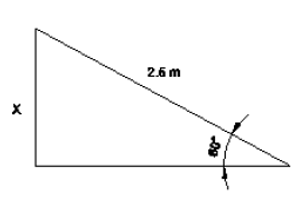top of page

## Workshop Calculation and Science

#### Year 1 Module 101: Which is equal to sinq sin
A : Opposite Side/Hypotenuse
B : Hypotenuse/Opposite side

2: What is equal to cosq  cos

C : Opposite side/Hypotenuse
D : Hypotenuse/Opposite Side

3: What is equal to tanq tan
A : Opposite Side/Hypotenuse

4: What is the value of q if sinq = Ö3/2 ?
A : 30°
B : 45°

C : 60°
D : 90°

5: What is 1 + cot2q  1 + cot2
A : sec2q

B : cosec2q
C : cot2q
D : tan2q

6: What is the height of the wall where the ladder touches the wall if the ladder is 2.5 m long makes an angle of 60° with the ground

A : 4.13 m
B : 4.23 m

C : 2.165 m
D : 4.43 m

7: What is the height of AC

A : 1.732 m
B : 17.32 m

C : 173.2 m
D : 1732 m

8: What is the height of the building if a ladder at 45° touches the building placed 16 m from the base of the building
A : 15 m

B : 16 m
C : 17 m
D : 18 m

9: What is the angle of elevation of the top of a light house of 15 m height seen at a point 15 m away from the base

A : 30°
B : 45°
C : 60°
D : 90°

10: What is the angle of q

A : 30°
B : 45°

C : 60°
D : 90°

11: What is the term for the object seen higher than eye level
A : Angle of inclination
B : Angle of friction

C : Angle of elevation
D : Angle of depression

bottom of page